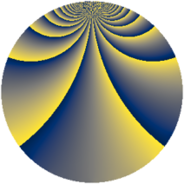# Properties

 Label 1045.2.bhLevel $1045$ Weight $2$ Character orbit 1045.bh Rep. character $\chi_{1045}(26,\cdot)$ Character field $\Q(\zeta_{15})$ Dimension $640$ Sturm bound $240$

# Related objects

## Defining parameters

 Level: $$N$$ $$=$$ $$1045 = 5 \cdot 11 \cdot 19$$ Weight: $$k$$ $$=$$ $$2$$ Character orbit: $$[\chi]$$ $$=$$ 1045.bh (of order $$15$$ and degree $$8$$) Character conductor: $$\operatorname{cond}(\chi)$$ $$=$$ $$209$$ Character field: $$\Q(\zeta_{15})$$ Sturm bound: $$240$$

## Dimensions

The following table gives the dimensions of various subspaces of $$M_{2}(1045, [\chi])$$.

Total New Old
Modular forms 992 640 352
Cusp forms 928 640 288
Eisenstein series 64 0 64

## Trace form

 $$640 q + 4 q^{2} - 4 q^{3} + 80 q^{4} + 6 q^{6} - 24 q^{8} + 68 q^{9} + O(q^{10})$$ $$640 q + 4 q^{2} - 4 q^{3} + 80 q^{4} + 6 q^{6} - 24 q^{8} + 68 q^{9} - 16 q^{10} + 8 q^{11} + 24 q^{12} + 8 q^{13} + 22 q^{14} + 80 q^{16} + 36 q^{17} - 36 q^{18} - 14 q^{19} - 10 q^{22} - 32 q^{23} + 34 q^{24} + 80 q^{25} - 40 q^{26} - 28 q^{27} + 30 q^{29} - 32 q^{30} - 16 q^{31} - 72 q^{32} + 46 q^{33} + 36 q^{34} - 4 q^{35} + 78 q^{36} - 32 q^{37} - 18 q^{38} - 36 q^{39} + 12 q^{40} - 10 q^{41} + 38 q^{42} + 24 q^{43} - 6 q^{44} + 72 q^{46} - 16 q^{47} + 46 q^{48} - 212 q^{49} - 8 q^{50} + 2 q^{51} + 22 q^{52} - 24 q^{53} - 112 q^{54} - 232 q^{56} - 54 q^{57} - 104 q^{58} - 6 q^{59} - 48 q^{61} + 80 q^{62} + 40 q^{63} - 232 q^{64} + 80 q^{65} + 18 q^{66} - 12 q^{67} - 184 q^{68} - 140 q^{69} + 36 q^{70} - 4 q^{71} + 112 q^{72} - 60 q^{73} - 32 q^{74} - 12 q^{75} + 140 q^{76} + 28 q^{77} + 40 q^{78} - 36 q^{79} - 24 q^{80} + 90 q^{81} - 70 q^{82} - 24 q^{83} - 8 q^{84} - 16 q^{86} - 160 q^{87} - 64 q^{88} + 48 q^{89} + 4 q^{90} - 30 q^{91} + 64 q^{92} + 144 q^{94} + 54 q^{97} - 188 q^{98} + 68 q^{99} + O(q^{100})$$

## Decomposition of $$S_{2}^{\mathrm{new}}(1045, [\chi])$$ into newform subspaces

The newforms in this space have not yet been added to the LMFDB.

## Decomposition of $$S_{2}^{\mathrm{old}}(1045, [\chi])$$ into lower level spaces

$$S_{2}^{\mathrm{old}}(1045, [\chi]) \cong$$ $$S_{2}^{\mathrm{new}}(209, [\chi])$$$$^{\oplus 2}$$